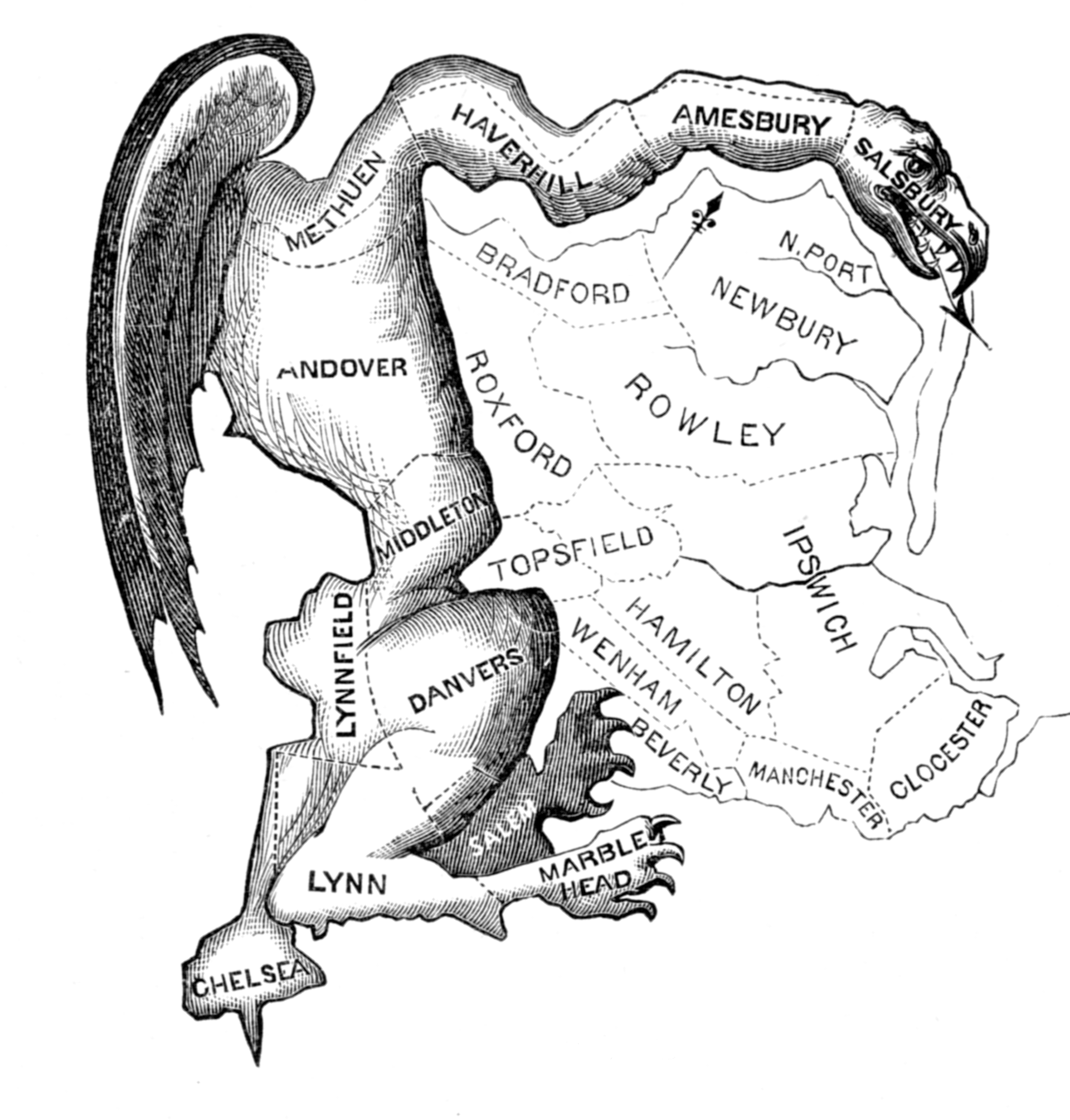## non-reversible gerrymandering

Posted in Books, Statistics, Travel, University life with tags , , , , , , , on September 3, 2020 by xi'anGregory Herschlag, Jonathan C. Mattingly [whom I met in Oaxaca and who acknowledges helpful conversations with Manon Michel while at CIRM two years ago], Matthias Sachs, and Evan Wyse just posted an arXiv paper using non-reversible MCMC methods to improve sampling of voting district plans towards fighting (partisan) Gerrymandering. In doing so we extend thecurrent framework for construction of non-reversible Markov chains on discrete samplingspaces by considering a generalization of skew detailed balance. Since this means sampling in a discrete space, the method using lifting. Meaning adding a dichotomous dummy variable, “based on a notion of flowing the center of mass of districts along a defined vector field”. The paper is quite detailed about the validation and the implementation of the method. With this interesting illustration for the mixing properties of the different versions:## Le Monde puzzle [open problem]

Posted in Books, Kids with tags , , , , , on October 23, 2017 by xi'anWhat should have been the last puzzle in Le Monde competition turned out to be an anticlimactic fizzle on how many yes-no questions are needed to identify an integer between 1 and 1025=2¹⁰+1 and an extension to replies possibly being lies

What is much more exciting is that voting puzzle #1021 got cancelled because the authors of this puzzle thought the cascading majority rule would produce the optimal solution and it does not! (As exhibited by my R code.) So here is an open problem to ponder about! (And another puzzle in the pipeline to complete the competition.)

## Le Monde puzzle [#838]

Posted in Books, Kids, R with tags , , , , , , , , , , on November 2, 2013 by xi'anAnother one of those Le Monde mathematical puzzles which wording is confusing to me:

The 40 members of the Academy vote for two prizes. [Like the one recently attributed to my friend and coauthor Olivier Cappé!] Once the votes are counted for both prizes, it appears that the total votes for each of the candidates take all values between 0 and 12. Is it possible that two academicians never pick the same pair of candidates?

I find it puzzling… First because the total number of votes is then equal to 78, rather than 80=2 x 40. What happened to the vote of the “last” academician? Did she or he abstain? Or did two academicians abstain on candidates for only one prize each?  Second, because of the incertitude in the original wording: can we assume with certainty that each integer between 0 and 12 is only taken once? If so, it would mean that the total number of candidates to the prizes is equal to 13. Third, the question seems unrelated with the “data”: since sums only are known, switching the votes of academicians Dupond and Dupont for candidates Durand and Martin in prize A (or in prize B) does not change the number of votes for Durand and Martin.

If we assume that each integer between 0 and 12 only appears once in the collection of the sums of the votes and that one academician abstained on both prizes, the number of candidates for one of the prizes can vary between 4 and 9, with compatible solutions provided by this R line of code:

```N=5
ok=TRUE
while (ok){
prop=sample(0:12,N)
los=(1:13)[-(prop+1)]-1
ok=((sum(prop)!=39)||(sum(los)!=39))}
```

which returns solutions like

```> N=5
> prop
  9 11  7 12
> los
  0  1  2  3  4  5  6  8 10
```

but does not help in answering the question!

Now, with Robin‘s help, (whose Corcoran memorial prize I should have mentioned in due time!), I reformulate the question as

The 40 members of the Academy vote for two prizes. Once the votes are counted for both prizes, it appears that all values between 0 and 12 are found among the total votes for each of the candidates. Is it possible that two academicians never pick the same pair of candidates?

which has a nicer solution: since all academicians have voted there are two extra votes (40-38), meaning either twice 2 or thrice 1. So there are either 14 or 15 candidates ex toto.  With at least 4 for a given prize. I then checked whether or not the above event could occur, using the following (pedestrian) R code:

```for (t in 1:10^3){
#pick number of replicae
R=sample(1:2,1); cand=13+R
#pick number of literary candidates
N=sample(4:(cand-4),1)
if (R==2){
}else{
ok=TRUE
while (ok){
drop=sample(1:cand,N)
ok=((sum(prop)!=40)||(sum(los)!=40))
}
pool=NULL
for (j in 1:N)
pool=c(pool,rep(j,prop[j]))
cool=NULL
for (j in 1:(cand-N))
cool=c(cool,rep(100+j,los[j]))
cool=sample(cool) #random permutation